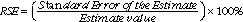1332.0.55.002 - Statistical Language!, 2008
ARCHIVED ISSUE Released at 11:30 AM (CANBERRA TIME) 27/06/2008  First Issue
Page tools:Print All

CALCULATIONS

Standard Deviation

The following example is a calculation of the standard deviation of the salaries of persons employed in The Widget Company.

 Employment details of 'The Widget Company'Observation Position in company Salary (\$)1 CEO 100 0002 Manager 60 0003 Manager 60 0004 Factory Worker 50 0005 Factory Worker 50 0006 Factory Worker 50 0007 Factory Worker 50 0008 Trainee 40 0009 Trainee 40 000The are six steps to calculating the standard deviation:

1. Calculate the mean

The mean of the salaries in the above table is \$55,555

2. Subtract the mean from each observation

This has been calculated in the above table under the heading 'Difference'. For example, the difference from the mean for the CEO's salary is 100,000 minus 56,555 which equals 44,444.

3. Square each result

This has been calculated in the above table under the heading 'Difference Squared'. For example the difference squared for the CEO's salary is 44,444 times 44,444 which equals 1,975,308,247.

In the example this is 1,975,308,246 + 19,753.047 + 19,753.047 + 30,864,247 + 30,864,247 +30,864,247 + 30,864,247 + 241,975,447 + 241,975,447 = 2,622,222,222.

5. Divide this sum by the number of observations (the result at this stage is called the "variance").

In this example this is 2,622,222,222 divided by nine (number of observations) which equals 291,358,024.69.

6. Take the positive square root

In this example the square root of 291,358,024.69 is 17,069.21. Therefore, the standard deviation is 17,069.21.

Standard Error

The standard error of the mean of a sample from a population is the standard deviation of the sample divided by the square root of the sample size.

Relative Standard Error

The RSE is generally calculated by with the following formula.Previous Page Next Page